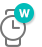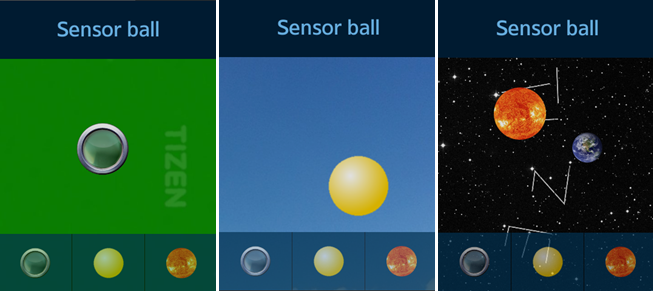# Sensor Ball Sample OverviewRelated Info

The Sensor Ball sample application demonstrates how you can create an application that simulates behavior of a ball in three different environments.

The user can impact the movement of the ball by moving the device. The application reads acceleration of the device and changes the position of the ball to simulate inertia and gravity forces.

The following figure illustrates the main screen of the Sensor Ball.

Figure: Sensor Ball screenThe main page of the application contains the scene and three buttons at the bottom. The buttons allow switching between the following environments: gravity, sky, and space.

• The gravity environment simulates a ball in indoor conditions. The movement of the ball is affected by gravity. The screenshot on the left presents the scene with this environment.

• The sky environment simulates a balloon in the sky. The balloon is filled with gas lighter than air, so it slowly moves up. The screenshot in the middle presents the scene.

• The space environment simulates a planet in space. Sun and Earth are placed on the scene and Earth orbits the sun as shown in the screenshot on the right. In this environment the gravity does not depend on acceleration of the device. Instead, positions of the Sun and the background are slightly changed in response to the device movement.

## Source Files

You can create and view the sample application project including the source files in the IDE.

File name Description
config.xml This file contains the application information for the platform to install and launch the application, including the view mode and the icon to be used in the device menu.
css/ This directory contains the CSS styling for the application UI.
images/ This directory contains the images used to create the user interface.
index.html This is a starting file from which the application starts loading. It contains the layout of the application screens.
js/app.js This file defines the initialization module.
js/core/ This file contains the application framework.
js/models/ball.js This file is the model of the ball. It contains the ball position and speed.
js/models/gravity.js This file is the model of the gravity. It performs calculations for the ball in the gravity mode.
js/models/space.js This file is the model of space. It performs calculations for the planet in space.
js/models/rectangular.js This file is the model of the rectangular scene. It calculates the collisions between the ball and the scene edges.
js/models/circular.js This is the model of a circular scene. It calculates the collisions between the ball and the scene edge.
js/views/ This directory contains the code to render the elements of the scene and handles the UI events.

## Implementation

The following functions demonstrate adding the devicemotion event listener and handling the event.

```/* views/main.js */
function onDeviceMotion(event)
{
ball.setMotionData(event);
}

function bindEvents()
{
var gravityButton = document.getElementById(GRAVITY_BUTTON_ID),
skyButton = document.getElementById(SKY_BUTTON_ID),
spaceButton = document.getElementById(SPACE_BUTTON_ID);

/* devicemotion event listener */

{
startGravity();
});

{
startSky();
});

startSpace();
});
```

The following function sets the motion data with the given event value.

```/* models/ball.js */
function setMotionData(event)
{
motionData = event;
}
```

The following function calculates the ball's velocity based on the sensor motion data.

```/* models/gravity.js */
function calculateVelocity()
{
var x = 0,
y = 0,
ddx = 0,
ddy = 0;

if (motionData !== null)
{
x = -motionData.accelerationIncludingGravity.x;
y = -motionData.accelerationIncludingGravity.y;
}
ddx = x * -cdd;
ddy = y * cdd;
v.x += ddx;
v.y += ddy;
v.x *= friction;
v.y *= friction;
}
```

The following function calculates the position of the Sun in the space mode based on the data received from the motion sensor.

```/* models/space.js */
function getSunPosition()
{
var result = {},

x = -motionData.accelerationIncludingGravity.x,
y = -motionData.accelerationIncludingGravity.y,

rX = -8.0,
rY = -8.0,

cX = (gameWidth - sunWidth) / 2,
cY = (gameHeight - sunHeight) / 2,

tX = cX + (-x * rX),
tY = cY + (y * rY),

bdX = tX - sunX,
bdY = tY - sunY,

br = 0.2;

sunX += bdX * br;
sunY += bdY * br;

result.x = sunX;
result.y = sunY;

return result;
}
```

The following function calculates the position of the background in the space mode based on the data received from the motion sensor.

```/* models/space.js */
function getBackgroundPosition()
{
var result = {},

x = -motionData.accelerationIncludingGravity.x,
y = -motionData.accelerationIncludingGravity.y,

rX = -30.0,
rY = -30.0,

cX = (gameWidth - backgroundWidth) / 2,
cY = (gameHeight - backgroundHeight) / 2,

tX = cX + (-x * rX),
tY = cY + (y * rY),

bdX = tX - backgroundLeft,
bdY = tY - backgroundTop,

br = 0.2;

backgroundLeft += bdX * br;
backgroundTop += bdY * br;

result.left = backgroundLeft;
result.top = backgroundTop;

return result;
}
```